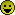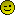Engine Management eCtune , Crome , AFC , FMU , Zdyne , Hondata , EMS , TE , Uberdata Anything to control your fuel, spark needs!

what do the fuel multipliers do?

1.0 BAR

Join Date: Nov 2004
Posts: 319what do the fuel multipliers do?

as the title suggests what does the fuel multipliers do? difference between enabled and disabled?3.0 BAR

Join Date: Apr 2003
Posts: 2,260Re: what do the fuel multipliers do?

1) Fuel multipliers are not "Enabled/Disabled". The option in TurboEDIT GUI is for auto-detection of fuel multipliers. Basically, so TurboEDIT will calculate these multipliers on it's own without you having to know what they are.

2) Here is the explaination I wrote on PGMFI to the same question..

--- begin cut ---

Multipliers are easy to understand..

Think of it like this, the ECU can only calculate 8-bit values 0-255 (0x00 - 0xFF).

The fuel multipliers give the ECU a way to calculate outside that value range.

For example, say we have a fuel multiplier of 0 representing no multiplier at all (in an example equation).

10 * 0 + 10 = 10

Assuming 10 is the "max" value we could use in the ECU, we add a multiplier.

10 * 1 + 10 = 20..
10 * 2 + 10 = 30..

See how the same "max value" is able to be used, but the use of a multiplier lets us get higher values?

Same goes with the fuel mutlipliers. Simply put, they allow us to use higher values in the ECU for the pulse durations.

______ end cut -----3.0 BAR

Join Date: Apr 2003
Posts: 11,059Re: what do the fuel multipliers do?

i think he meant to say fuel 'modifiers'3.0 BAR

Join Date: Apr 2003
Posts: 2,260Re: what do the fuel multipliers do?

TurboEDIT doesn't have fuel modifers. It has Fuel Multipliers. :P3.0 BAR

Join Date: Apr 2003
Posts: 11,059Re: what do the fuel multipliers do?

Originally Posted by TurboEF9
TurboEDIT doesn't have fuel modifers. It has Fuel Multipliers. :P
i know what multipliers areuberdata and crome have fuel modifiers that you can enable/disable3.0 BAR

Join Date: Apr 2003
Posts: 11,059Re: what do the fuel multipliers do?

you don't see the multipliers in the program3.0 BAR

Join Date: Apr 2003
Posts: 2,260Re: what do the fuel multipliers do?

Ya well, he's talking about TurboEDIT. :P Get that OBD1 crap out of here.3.0 BAR

Join Date: Apr 2003
Posts: 2,260Re: what do the fuel multipliers do?

you know, youre right ghetto, im sorryShow Printable VersionEmail this Page